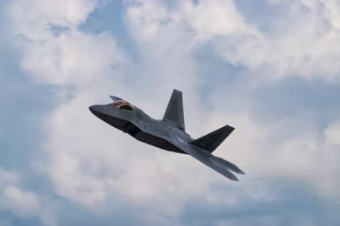## Fighter Jet

Consider a fighter jet is flying through 15°C air at 2,000 km/hr. What is its Mach Number if the air’s ratio of specific heats is 1.7? Assume the molecular weight of air is 29 kg/kmol, and note the universal gas constant is 8,314 J/(kmol·K).Hint
The Mach Number (Ma) is the ratio of fluid velocity to the speed of sound.
$$Ma=\frac{V}{c}$$$where $$V$$ is the mean fluid velocity, and $$c$$ is the local speed of sound. Hint 2 The local speed of sound in an ideal gas is: $$c=\sqrt{kRT}$$$
where $$k$$ is the ratio of specific heats, $$R$$ is the specific gas constant, and $$T$$ is the absolute temperature.
First, let’s find the local speed of sound in the ideal gas:
$$c=\sqrt{kRT}$$$where $$k$$ is the ratio of specific heats, $$R$$ is the specific gas constant, and $$T$$ is the absolute temperature. To find $$R$$ : $$R=\frac{\bar{R}}{(mol.wt_i)}$$$
where $$\bar R$$ is the universal gas constant. Thus,
$$R=\frac{8,314J(kmol)}{(kmol\cdot K)(29kg)}=286.69\frac{J}{kg\cdot K}=286.69\:\frac{m^2}{s^2\cdot K}$$$The local speed of sound in air is: $$c=\sqrt{(1.7)(286.69\frac{m^2}{s^2\cdot K})(15+273K)}=\sqrt{(487.4)(288)m^2/s^2}=374.65\:\frac{m}{s}$$$
The Mach Number (Ma) is the ratio of fluid velocity to the speed of sound:
$$Ma=\frac{V}{c}$$$where $$V$$ is the mean fluid velocity, and $$c$$ is the local speed of sound. Finally, $$Ma=\frac{2,000\frac{km}{hr}\cdot 1,000\frac{m}{km}}{374.65\frac{m}{s}\cdot 3,600\frac{s}{hr}}=\frac{2,000,000m/hr}{1,348,740m/hr}=1.48=1.5$$$
1.5